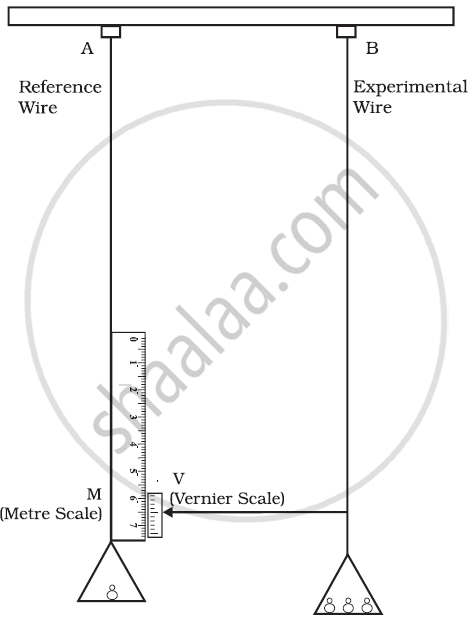Share

# Elastic Moduli - Determination of Young’s Modulus of the Material of a Wire

Course

#### notes

Determination of Young’s Modulus of the Material of a Wire:• Two strings were hung from a support and two pans were attached to both the strings.

• Weights are kept on both the pans.

• When the number of weights in the second pan was increased, the string got stretched and moved in a downward direction.

• The change in length was measured by the metre scale which was kept on reference wire.

• Using this experiment, the Young’s modulus value was calculated

• "Y"= "longitudinal stress"/ "longitudinal strain" = σ/ ε

• = ("F"/"A")/ ("ΔL"/"L")

• Where original length = L and ΔL = change in length, F=mg (acting downwards) and A (area of cross-section of wire) = pir^2

• = ("mg"/ (πr^2))/ (Δ"L"/"L")

• Y="mgL"/(pi"r"^2Delta"L")

• This is the way to calculate the Young’s modulus.

If you would like to contribute notes or other learning material, please submit them using the button below.